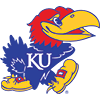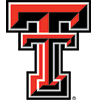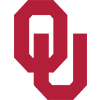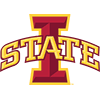DraftKings Big 12 Odds
+180
1.8 to 1Baylor
28.6% implied probability

+180
1.8 to 1Kansas
28.6% implied probability

+430
4.3 to 1Texas
15.1% implied probability

+445
4.45 to 1West Virginia
14.7% implied probability

+625
6.25 to 1Texas Tech
11.0% implied probability

+10000
100 to 1Oklahoma
0.8% implied probability

+15000
150 to 1Iowa State
0.5% implied probability

+20000
200 to 1TCU
0.4% implied probability

+30000
300 to 1Kansas State
0.3% implied probability

No changes have been recorded yet.